Finding sum from nth number

Chapter 8 Class 11 Sequences and Series
Concept wise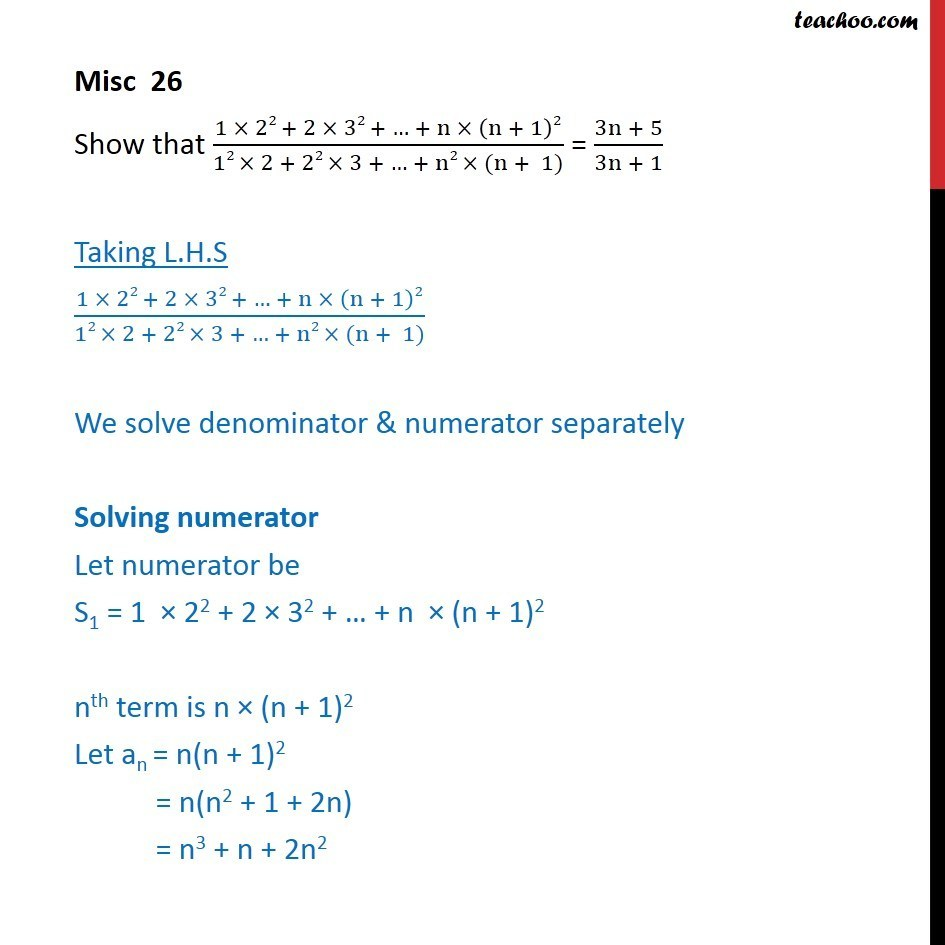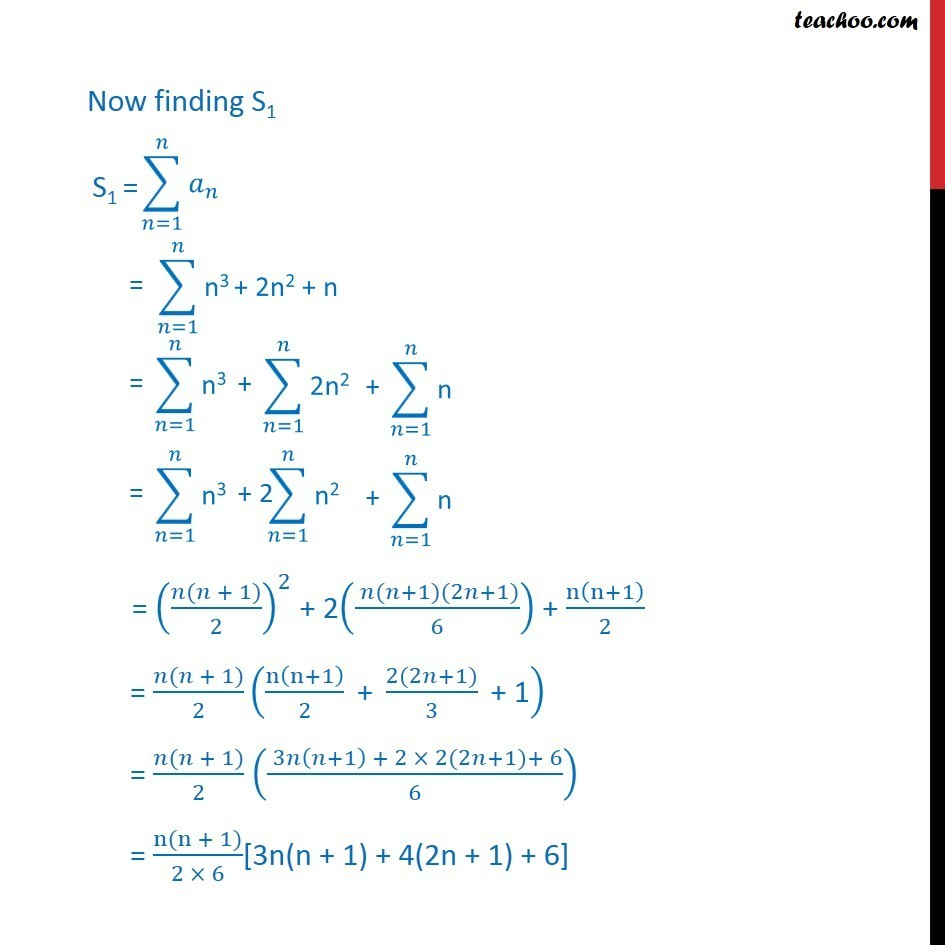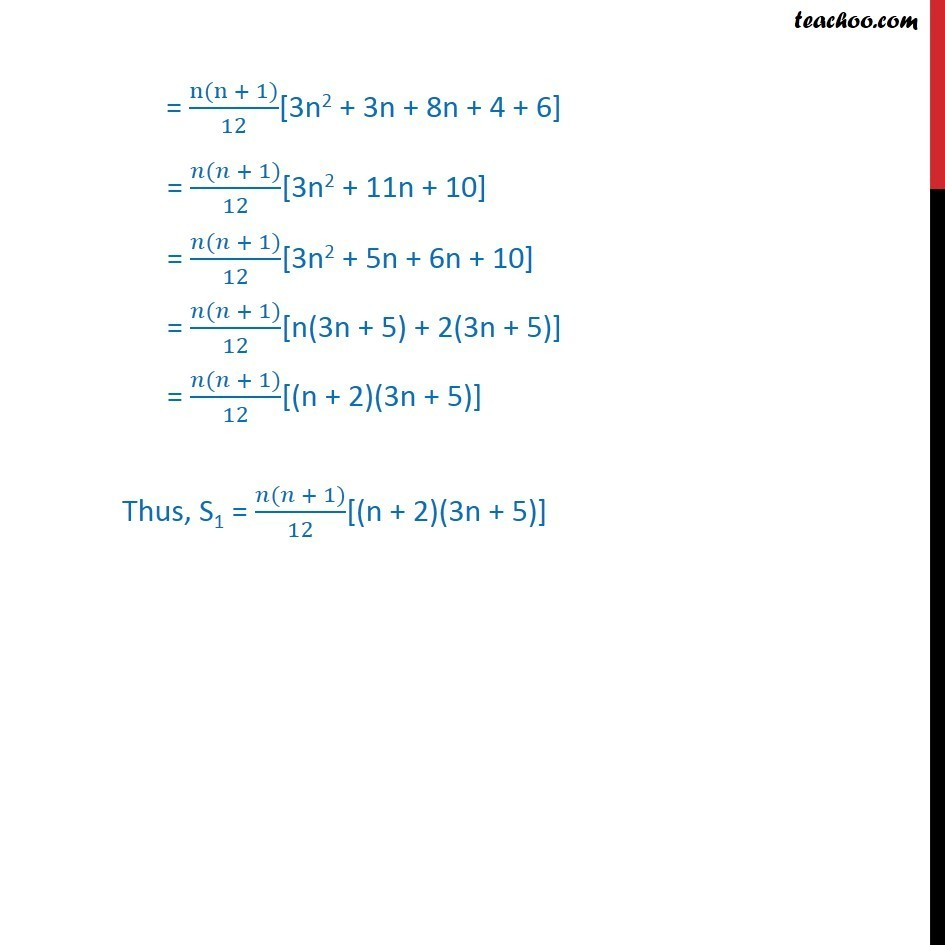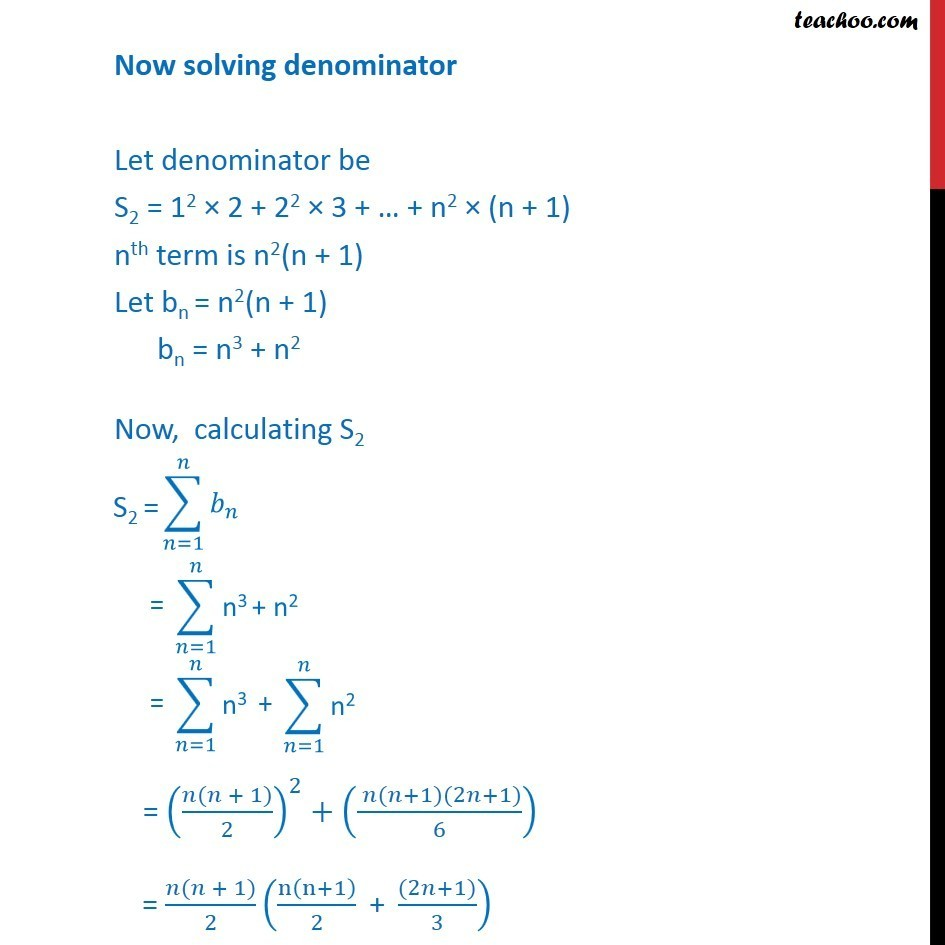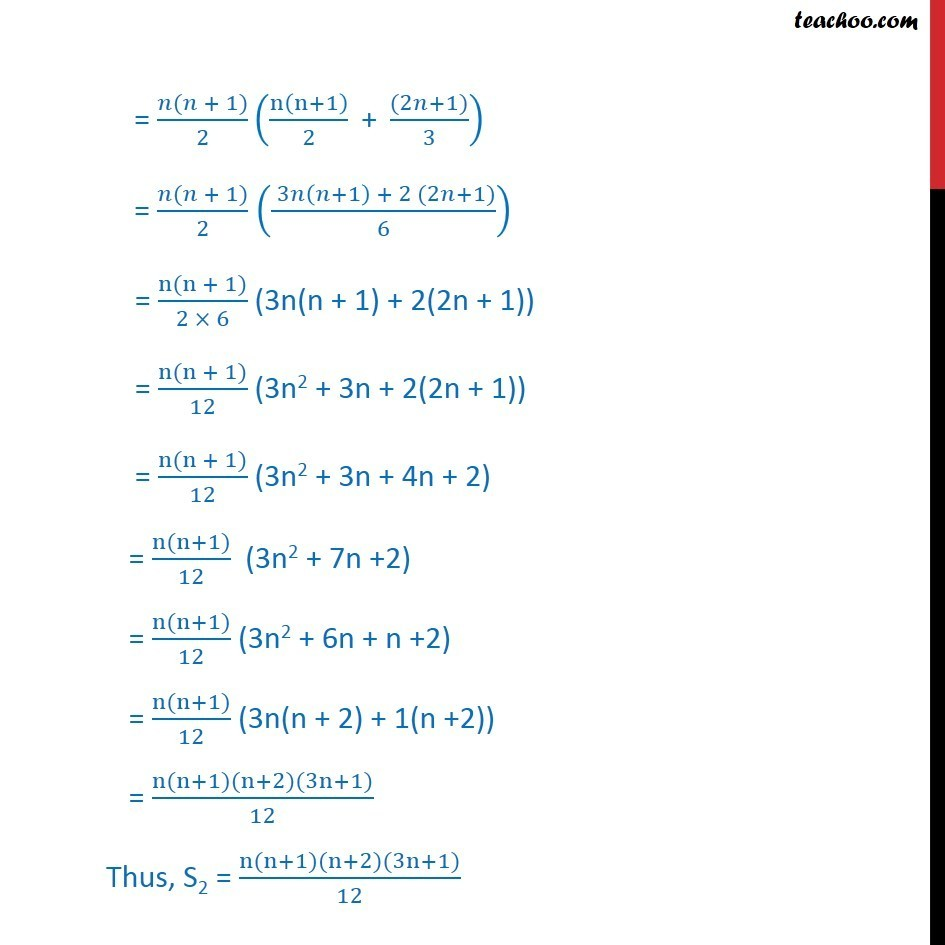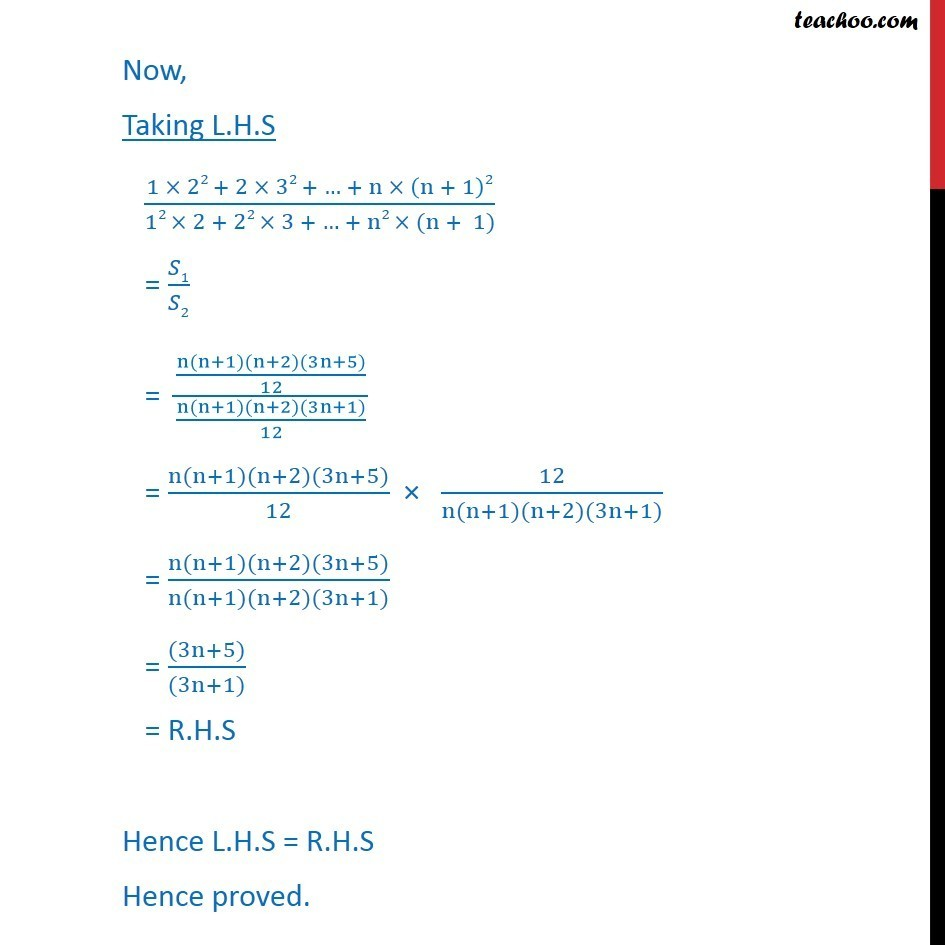Learn in your speed, with individual attention - Teachoo Maths 1-on-1 Class

### Transcript

Misc 26 Show that (1 22 + 2 32 + + n (n + 1)2)/(12 2 + 22 3 + + n2 (n + 1)) = (3n + 5)/(3n + 1) Taking L.H.S (1 22 + 2 32 + + n (n + 1)2)/(12 2 + 22 3 + + n2 (n + 1)) We solve denominator & numerator separately Solving numerator Let numerator be S1 = 1 22 + 2 32 + + n (n + 1)2 nth term is n (n + 1)2 Let an = n(n + 1)2 = n(n2 + 1 + 2n) = n3 + n + 2n2 Now finding S1 = (( ( + 1))/2)^2 + 2(( ( +1)(2 +1))/6) + n(n+1)/2 = ( ( + 1))/2 (n(n+1)/2 " + " (2(2 +1))/3 " + 1" ) = ( ( + 1))/2 (( 3 ( +1) + 2 2(2 +1)+ 6)/6) = (n(n + 1))/(2 6)[3n(n + 1) + 4(2n + 1) + 6] = (n(n + 1))/12[3n2 + 3n + 8n + 4 + 6] = ( ( + 1))/12[3n2 + 11n + 10] = ( ( + 1))/12[3n2 + 5n + 6n + 10] = ( ( + 1))/12[n(3n + 5) + 2(3n + 5)] = ( ( + 1))/12[(n + 2)(3n + 5)] Thus, S1 = ( ( + 1))/12[(n + 2)(3n + 5)] Now solving denominator Let denominator be S2 = 12 2 + 22 3 + + n2 (n + 1) nth term is n2(n + 1) Let bn = n2(n + 1) bn = n3 + n2 Now, calculating S2 = (( ( + 1))/2)^2 + (( ( +1)(2 +1))/6) = ( ( + 1))/2 (n(n+1)/2 " + " ((2 +1))/3) = ( ( + 1))/2 (n(n+1)/2 " + " ((2 +1))/3) = ( ( + 1))/2 (( 3 ( +1) + 2 (2 +1))/6) = (n(n + 1))/(2 6) (3n(n + 1) + 2(2n + 1)) = (n(n + 1))/12 (3n2 + 3n + 2(2n + 1)) = (n(n + 1))/12 (3n2 + 3n + 4n + 2) = (n(n+1))/12 (3n2 + 7n +2) = (n(n+1))/12 (3n2 + 6n + n +2) = (n(n+1))/12 (3n(n + 2) + 1(n +2)) = (n(n+1)(n+2)(3n+1))/12 Thus, S2 = (n(n+1)(n+2)(3n+1))/12 Now, Taking L.H.S (1 22 + 2 32 + + n (n + 1)2)/(12 2 + 22 3 + + n2 (n + 1)) = 1/ 2 = ((n(n+1)(n+2)(3n+5))/12)/((n(n+1)(n+2)(3n+1))/12) = (n(n+1)(n+2)(3n+5))/12 12/(n(n+1)(n+2)(3n+1)) = (n(n+1)(n+2)(3n+5))/(n(n+1)(n+2)(3n+1)) = ((3n+5))/((3n+1)) = R.H.S Hence L.H.S = R.H.S Hence proved.